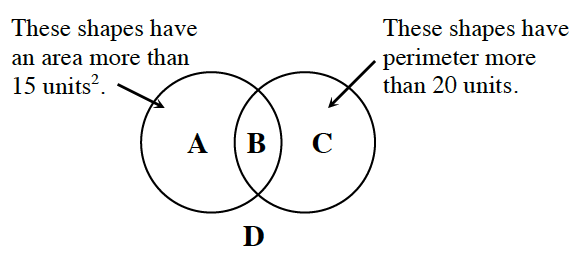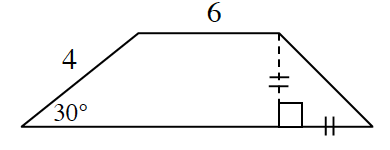### Home > CCG > Chapter 11 > Lesson 11.1.3 > Problem11-46

11-46.

Examine the Venn diagram at right. In which region should the figure below be placed? Show all work to justify your conclusion.Divide the trapezoid into portions and use special right triangles to help find side lengths.

The formula to find the area of a trapezoid is: $\frac{1}{2}(\text{base}_1+\text{base}_2)(\text{height})$

If area $≈ 17.46\text{ units}^2$ and perimeter $≈ 24.3$ units, where should the figure be placed?